telescopeѲptics.net          ▪▪▪▪                                             CONTENTS

5.1.2. seeing error: limiting resolution, Strehl, otf

Due to the random nature of seeing-induced error, the Strehl approximation for non-random aberrations, given by Eq. 56 becomes inaccurate for larger errors, considerably more than it is for conventional aberrations (FIG. 97). The effect could be mainly due to the roughness error component, whose increase reduces the average relative size of wavefront irregularity, while at the same time increasing its average RMS error. Similarly to ordinary surface roughness, narrow zones and turned edge, the increase in the P-V (and thus RMS) error after a certain point begins to drain less energy out of the Airy disc, with the main effect gradually becoming merely spreading the energy out wider.

As a result, the increase in the RMS (surface or wavefront) error is followed by a relatively slower Strehl degradation, as given by the relation derived by Racine (The Telescopic Point-Spread Function, 1996; its general form is given on FIG. 83):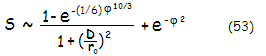e being the natural logarithm base (e~2.718, rounded off to three decimals) and φ2 the variance over the pupil. For long-exposure, φ2=1.03(D/r0)5/3, and for short exposure φ2=0.132(D/r0)5/3 (note that the constant value here is slightly different than 0.134 with Mahajan).

Variance is directly related to the standard deviation, i.e. the RMS error. If φ2=(2πφL)2 is the phase variance across the pupil, then φL=0.162(D/r0)5/6 amounts to the time-averaged (long-exposure, defined as an exposure significantly longer than the coherence time) RMS wavefront error induced by seeing (Eq. 52.1). So, for D/r0=1, the average RMS wavefront error φL=0.162, giving φ2=1.03, and the seeing Strehl S=0.445; for the nominally identical linear RMS wavefront error ω of non-random aberrations the Strehl (from Mahajan's approximation) is 0.357.

For short exposure (at the level of coherence time, or shorter), the corresponding RMS wavefront error is φL=0.0582(D/r0)5/6 (Eq. 52.2).

A simpler long-exposure Strehl approximation, for (D/r0)~1 and larger, is S~1/[1+1.23(D/r0)2], and even simpler, for (D/r0) values over 5, is S~1/(D/r0)2. A quick long exposure seeing Strehl approximation, empirically fitted for (D/r0) around 1 and smaller is S~1-0.55(D/r0).

The exact Strehl values from the Racine's paper are given in the following table.

 SEEING STREHL RATIOS FOR LONG (SL) AND SHORT EXPOSURES (SS) D/r0 SL SS SS/SL 0.0 1.000 1.000 1.00 0.1 0.978 0.997 1.02 0.5 0.741 0.948 1.28 1 0.445 0.844 1.90 2 0.175 0.594 3.38 3 0.089 0.369 4.17 3.5 0.067 0.285 4.23 4 0.053 0.218 4.10 5 0.035 0.128 3.64 7 0.019 0.051 2.76 10 0.009 0.021 2.18

Note that the calculations are based on the standard Kolmogorov turbulence model, which assumes an infinite outer scale of turbulence. While the paper claims good agreement with experimental data, it is to expect that these numbers are generally somewhat pessimistic, due to the error reduction resulting from the finite outer scale of turbulence in the actual field condition.

Due to increase of the effect of the tilt error component in visual observing, either with the increase in aperture (for given seeing level), or in the seeing error (for given aperture), the resulting visual Strehl gradually shifts from being near identical to the short-exposure Strehl (for D/r0~1, or smaller, such as in very small apertures in the average or better seeing, or small-to-medium apertures in exceptionally good seeing), to be closer to the long-exposure Strehl (for D/r0~5 and larger seeing errors).

The rate of gradual incerase in the proportion of tilt error in the total error is unknown, at least I'm not aware of any theoretical, or practical concept of it. Nominally, it would represent a gradual increase from the RMS error for wavefront roughness alone (short-exposure error), RMS(r)=0.058(D/r0)5/6, toward which the total error gravitates for D/r0<1, to the full-blown long-exposure error, RMS(r+t)=0.0162(D/r0)5/6, that includes both, full extent of tilt and roughness, toward which gravitates the error for D/r0 >5. This transition can be illustrated with application of a simple arbitrary factor, as shown below.Dashed red line reflects gradual increase in the proportion of the tilt error with the increase in D/r
0. While the rate of increase shown is entirely arbitrary, it may serve as a visual reference of the approximate rate of change, and probably is a good first approximation of the actual rate.

Plot on FIG. 83 illustrates basic relations between the three main forms of seeing-induced error: long exposure, short exposure and visual.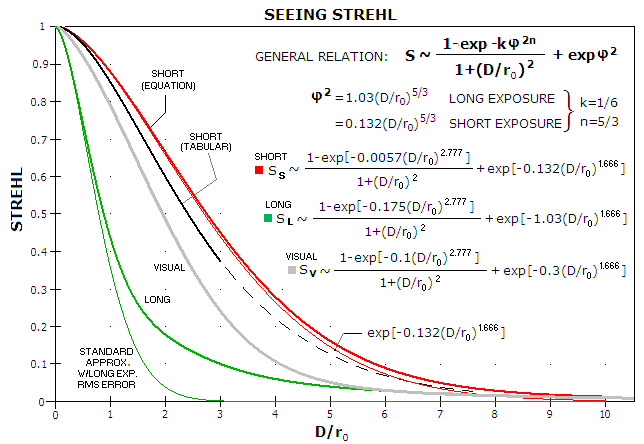FIGURE 83
: Strehl ratios for the wavefront error induced by atmospheric turbulence. For the ratio values below ~0.6, or long-exposure RMS wavefront error in units of the wavelength greater than ~0.1, the long-exposure Strehl (upper green) becomes increasingly better than what  the standard Strehl approximation ((lower green, Eq. 56) indicates for nominally identical RMS wavefront error of non-random aberration (note that the standard Strehl approximation is within 3, 10 and 20% from the true value for balanced primary spherical aberration at 0.5, 0.3 and 0.1 Strehl, respectively). Plot for the short-exposure Strehl is for the roughness error component alone, smaller by a factor of 0.37 than long-exposure RMS error. As a result, it decreases more slowly with the increase in seeing error than long-exposure Strehl. Visual Strehl as a function of D/r
0 is approximated so that it gradually shifts from being near equal to the short-exposure Strehl for D/r0~1 and smaller, to being nearly equal to the long exposure Strehl for D/r0 larger than 5. Accidently or not, it is at the lower diffraction limit (0.80) for D/r0=1, A simpler fit to this approximation for the visual Strehl is S=0.98exp[-0.16(D/r0)2]+0.02; it is a bit more optimistic for D/r0 of about 2 and smaller - for instance, would give ~0.85 for D/r0=1 - but overall close to the plot for D/r0<10 (for D/r0 smaller than ~3 it can be reduced to S=exp[-0.16(D/r0)2]). Note that exp[x] = ex.
The two plots for short exposure Strehl are due to an apparent error in Racine's paper. The short exposure plot as given in the paper (black, given for the D/r
0 range 0-3, with the dashed portion approximating its extension) cannot be obtained from the general form of the Strehl equation (top) as given in the paper, because the right term alone (lower red) plots higher, and the left term, even if generally small, is always positive, i.e. adds up. Till final clarification, either plot could be the correct one.

Standard approach to the seeing error is for long-exposure. In it, the size of r0 determines angular resolution of large telescopes as α~λ/r0 in radians, or α~206,265λ/r0 in arc seconds  (λ being the wavelength of light), as opposed to the "standardized" diffraction resolution given by ~λ/D. While the size of r0 results from a complex function, it can be expressed much more simply in terms of the resolution limit it imposes to large telescopes. For seeing imposed stellar resolution α in radians, and wavelength λ, it is approximated by:

r0 ~ λ/α            (54)

Hence, an average ~2 arc second seeing at the zenith, for λ=0.00055mm, results from r0~57mm (2 arc seconds in radians is 2/206,265). Note that the averaged turbulence limited stellar resolution is often cited as somewhat inferior to the above: α~1.2λ/r0, or α~1.27λ/r0 (Schroeder). Obviously, if these are taken to represent seeing, the value of r0 corresponding to the given nominal seeing figure will be proportionally larger as well.

Since r0 is changing in proportion to λ1.2 and (cosγ)0.6, γ being the zenith angle in degrees, a more complete expression for its size is given by: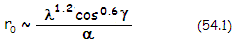Thus, the extracted limiting stellar resolution is: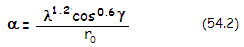where r0 is the atmospheric coherence length for λ=0.00055mm corresponding to the zenith angle γ.

So, for instance, at 30� from zenith (γ=30�) and the wavelength λ=0.0005mm, atmospheric coherence length r0=100mm for 0.00055mm wavelength results in r0'=(0.0005/0.00055)1.2(cos30)0.6=0.77r0=77mm coherence length for 0.0005mm wavelength. The corresponding limiting stellar resolution ("seeing") at this wavelength is α'~0.0005/77 radians, or 1.34 arc seconds (after multiplying by 206,265).

Following table gives rounded off values of long-exposure r0 and α in 2 arc seconds seeing for several values of zenith angle γ, for λ=0.00055mm. Values are calculated based on seeing-limited resolution given as α~λ/r0 in radians (α~206,265λ/r0 arc seconds); for seeing limited resolution given as α~1.2λ/r0 or α~1.27λ/r0, the atmospheric coherence length is larger by a factor equal to the numerical constant.

 Zenith angle γ (degrees) 0 15 30 45 60 75 Atmospheric coherence length r0 (mm) 57 55 50 40 29 15 Limiting stellar resolution α (arc seconds) 2 2.1 2.3 2.8 4 7.7

For small amateur telescopes over 60mm in aperture diameter, limiting visual stellar resolution for these r0 values will be generally better due to significantly smaller seeing-induced error level.

Linear long-exposure seeing diameter - an aspect of seeing-limited telescope resolution that can be important in imaging applications - is FWHML=α=λ�/r0=(D/r0)λF, with being the system focal length.

In the average 2 arc seconds seeing, with r0~57mm, a 300mm aperture with (D/r0) of ~5, the average long-exposure seeing FWHM diameter is about 5λF linearly, twice the Airy disc size, and larger than system's aberration-free FWHM (~λF) by a 1.2 D/r0 factor or, in this case, five times.

For short exposures (generally less than 1/20 of a second, specifically at the level of the coherence time, or smaller), the speckle movement caused by wavefront tilt error ceases, and both seeing blur and its FWHM shrink. If, for the purpose of obtaining a general idea of short-exposure FWHM, the tilt-component RMS wavefront error - from Eq. 52.1-2, rounded to 0.1(D/r0)5/6, in units of the wavelength - is assumed to be ~1/3 of the averaged P-V tilt error W on the wavefront, averaged angular displacement of the speckle structure due to wavefront tilt error is αt~Wλ/D~0.3(D/r0)5/6λ/D in radians. The corresponding average linear displacement from the center in the image plane is αt, being the focal length, or 0.3(D/r0)5/6λF. In other words, for given r0, nominal angular tilt error decreases relatively slowly - in proportion to D5/6/D=1/D1/6 - with the increase in aperture, but effectively increases relative to the angular size of diffraction pattern, in proportion to D/D1/6. For instance, nominal angular motion  of the image will be about 32% smaller in 100cm compared to 10cm aperture, but in terms of the ten times smaller angular pattern of the former, it will be 6.8 times larger. Nominal linear motion for given λF is proportional to (D/r0)5/6. or, for given r0, in proportion to D5/6.

Accordingly, linear diameter of the short-exposure FWHM is approximated by deducting 0.6(D/r0)5/6λ from long-exposure FWHM, i.e. FWHMS~(D/r0)λF-0.6(D/r0)5/6λF. Alternately, it is smaller than long-exposure FWHM by a factor 1-[0.3(D/r0)5/6/0.5(D/r0)]=1-0.6(D/r0)-1/6. This is only a crude approximation, producing FWHMS value for D/r0=1 well below λF - in part due to FWHML~(D/r0)λF underestimating FWHML value for D/r0 close to 1 - but it does indicate two important features of the seeing FWHM:

(1) short-exposure FWHM is nearly identical to the aberration-free FWHM for the low single-digit D/r0 values, and

(2) relative long-exposure enlargement of the short-exposure FWHM diminishes with D/r0 increase.

The variance of the image motion is given by Tyler as φ02=0.34(λ/r0)2(D/r0)-1/3 arcsec2, or as standard (RMS) deviation φ0=0.58(λ/r0)(D/r0)-1/6 arc seconds, where λ/r0 is the long-exposure seeing in radians (206,265λ/r0 in arc seconds). Gaussian approximation of the intensity distribution within the image (atmospheric PSF) is given by I~exp-0.5(θ0/φ0)2.

Following graph (based on Hardy, 1998) shows the actual relationship between long-exposure and short-exposure FWHM angular size, both normalized to λ/r0, as a function of D/r0. Thus, the diffraction-limited plot (blue) shows the size of diffraction-limited FWHM (/D) in units of λ/r0; since r0~D/x, x being the numerical value for D/r0, λ/r0~xλ/D.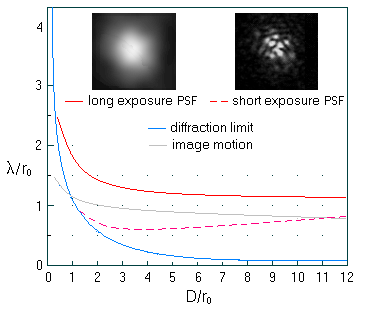FIGURE 84: The plots are showing that the seeing FWHM is significantly smaller for short vs. long exposure. The difference is largest at D/r0~1 or less, gradually diminishing toward larger D/r0 values. Limit to the long exposure resolution is generally somewhat larger than λ/r0, more in accord with 1.2λ/r0 or 1.27λ/r0 However, the specific value of the numerical portion varies with D/r0, being the highest for D/r0~1 (about 1.8), then quickly decreasing toward D/r0~2 (about 1.4), after which it slowly decreases toward 1 with higher D/r0 values. Note that 1.8λ/r0 long exposure FWHM for D/r0~1 (which equals 1.8λ/D, since r0~D) is significantly larger than in some other sources (Mahajan, Bass/DeCusatis, around 1.4λ/r0, following graph). Discrepancies are fairly common, reflecting complexity of the subject. Since the limiting resolution of ~1.8λ/D cannot be considered diffraction limited, this particular discrepancy may be result of a different modeling of the turbulence and/or exposure. Short exposure image becomes significantly larger than diffraction limit as D/r0 exceeds 2, which also indicates larger relative size compared to, for instance, Bass and DeCusatis, who show no appreciable effect on FWHM for this D/r0 value (following graph).

The relative image motion plot shows already mentioned tendency of subsiding with larger D/r0  values. However, it diminishes only in terms of λ/r0. The relative amplitude of image motion, in units of the actual FWHM, λ/D, steadily increases, from about 1 at D/r0=1 to about 8 at D/r0=10, and nearly in proportion to the change in D/r0 for its larger values. It is mainly caused by the increase in the tilt error component. The increased amplitude of image motion causes increased blurring of the visual image, as perceived by the eye.

Graph below shows how the averaged intensity distribution (PSF) for long and short exposure seeing PSF deteriorate with the increase in seeing error.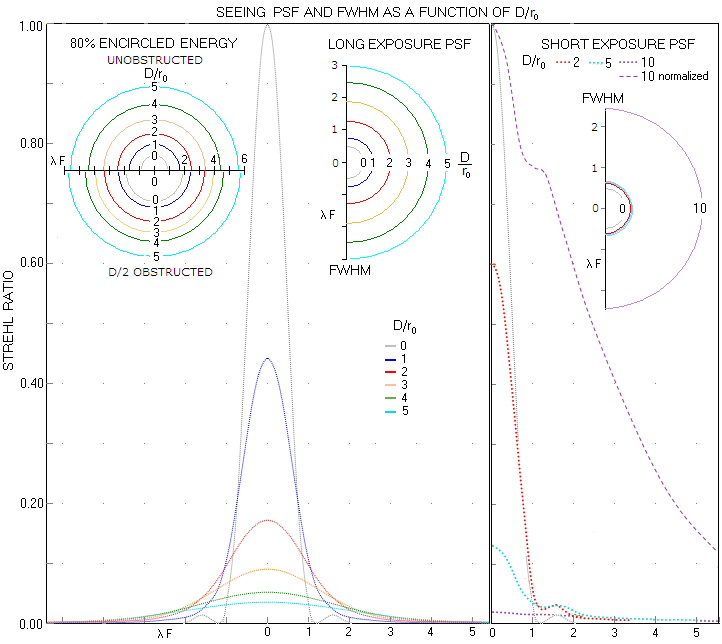FIGURE 85: LEFT: Long exposure seeing PSF for selected D/r0 values, relative to the PSF for D/r0=0 normalized to 1. RIGHT: Short-exposure seeing PSF for selected  D/r0 values (for clarity, plot for D/r0=10 is also shown normalized to 1). Note that these are PSF obtained under assumptions of the standard Kolmogorov model, specifically, an infinite outer scale of turbulence. The actual PSF are generally smaller for given D/r0 value, due to error reduction resulting from the finite (actual) outer scale.

With long exposure, ring structure is already lost at (D/r0)=1. The plot asymptotically approaches horizontal axis, thus can be approximated with simple Gaussian functions for any D/r0 value. After relatively small initial FWHM increase for (D/r0)~1 or smaller, its diameter further increases approximately by a factor of 1+(D/r0F, for (D/r0) values up to 10, and beyond.

Energy encircled within FWHM slowly diminishes from 50% of the total energy at (D/r0)=0 to 40% at (D/r0)=5. Loss of FWHM energy is less of a factor than its enlargement: at (D/r0)=5 the FWHM diameter is ten times larger than in aberration-free aperture. This results in as much inferior limiting stellar resolution. For unobstructed aberration-free aperture, radius of the 80% energy circle ranges from 0.9λF for (D/r0)=0 to 5.6λF for (D/r0)=5.

EFFECT OF CENTRAL OBSTRUCTION

Aberration-free aperture with D/2 central obstruction diameter is clearly inferior at (D/r0)=0, with the 80% energy radius of 1.9λF; as seeing error increases, the difference in the circle radius vs. unobstructed aperture diminishes, nearly disappearing at (D/r0)~5 and larger. Numerical aspect of the changes in FWHM and EE are given in table below.

 FWHM, STREHL AND 80% ENCIRCLED ENERGY RADIUS AS A FUNCTION OF D/r0 D/r0 0 1 2 3 4 5 FWHM (λF) UNOBSTRUCTED 0.5 0.75 1.3 1.6 2.1 2.6 D/2 C. OBSTRUCTION 0.47 0.7 1.3 1.8 2.25 2.75 STREHL (long exposure) UNOBSTRUCTED 1 0.445 0.175 0.089 0.053 0.035 D/4 C. OBSTRUCTION 1 0.430 0.169 0.088 0.054 0.036 D/2 C. OBSTRUCTION 1 0.391 0.160 0.090 0.058 0.040 80% EE (λF) UNOBSTRUCTED 0.9 1.6 2.5 3.4 4.4 5.6 D/2 C. OBSTRUCTION 1.9 2.4 3.3 3.9* 4.6* 5.7*

* estimate based on projecting plots beyond (D/r0)=3 in Optical Imaging and Aberrations 2, Mahajan (p418)

Consistent with its general effect, presence of central obstruction causes shift of energy outside of central maxima. Expectedly, the effect on PSF and encircled energy generally diminishes with the increase in seeing error (FIG. 86). In part, it is due to the long-exposure Strehl - the measure of wavefront quality, independent of the effect of central obstruction - becoming higher for the obstructed aperture as the size of obstruction and D/r0 ratio increase. The effect of obstruction on the Strehl is nearly neutral at (D/r0)~3, negative for smaller, and positive for larger ones. While seemingly contradictory, it could be rationalized by the aberration contribution from the area blocked by obstruction changing vs. that of the annulus area for with the change in (D/r0). For the lower values, i.e. for coherence length larger relative to the aperture, pupil area blocked by obstruction tends to have lower than average wavefront error, thus the annulus alone has it higher. For the smaller relative coherence lengths, however, pupil area blocked by obstruction begins to contribute more to the overall error (in unobstructed aperture), while the narrower annulus has more of the effect analogous to that of aperture reduction.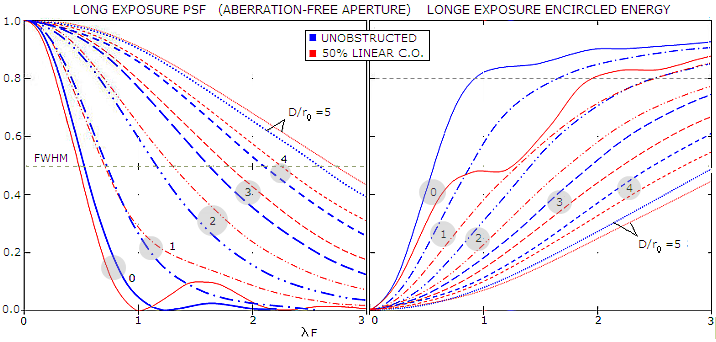FIGURE 86: The effect of 0.5D central obstruction on time-averaged long-exposure PSF (left) and encircled energy (right). Transfer of energy from central maxima to the outer portion of the central maxima persists as seeing error increases, but is quickly also compounded by the enlargement of the central maxima itself. Expectedly, encircled energy of the obstructed aperture is also lagging behind (source: Optical imaging and aberrations 2, Mahajan). As mentioned before, since these PSF are based on the assumption of an infinite outer scale of turbulence in the standard Kolmogorov model, they are generally somewhat pessimistic.

Short exposure PSF shows no significant FWHM increase even at (D/r0)=5; its intensity distribution retains some form of secondary maxima well beyond this D/r0 value. Its relative intensity of about 0.3 peak intensity means that it appears nearly half as bright to the eye. Since this intensity distribution is a product of significant wavefront roughness, the ring structure is broken into randomly moving segments, already resembling speckle structure.

In general, for achieving near-limiting resolution and contrast, pixel size shouldn't be larger than FWHM/2. This relative pixel size preserves limit to resolution set by FWHM, with pixel contrast transfer being only moderately lowered. For maximizing limiting magnitude, however, it should be somewhat larger than FWHM. A compromise pixel size is in between, depending on which of the two aspects is more important.

Even with pixel size optimized for contrast (i.e. not significantly larger than one half of the seeing FWHM), contrast transfer will be lowered due to the spread of energy caused by seeing error - the larger error, the more so.

A fairly popular concept in imaging is the probability of "lucky exposure", defined as a very short exposure (less than half coherence time) capturing moment of diffraction limited seeing, or better. Diffraction-limited is defined as 1 radian2 RMS phase variance, or less, which corresponds to 1/2π RMS, or less. For instantaneous exposure, this error level corresponds to (D/r0)~3.4. The original Fried's paper gives the following probabilities (P) for selected D/r0 values:

 D/r0 2 3 4 5 6 7 10 15 P 0.986 0.765 0.334 0.094 0.019 0.0029 1.1e-06 3.4x10-15

The paper also gives a fitted equation approximating P (whose exact value is given by a complex integral) as P~5.6exp[-0.1557(D/r0)2], where exp stands for the natural logarithm base e~2.72 under the exponent in brackets. It becomes progressively too optimistic for D/r0~6 and smaller, coming to P=1 for D/r0~3.35. For that reason, the paper states that it is valid for D/r0≥3.5.

The probability applies only for the area covered by isoplanatic angle (isoplanatic patch), usually less than 10 arc seconds in diameter. Significantly larger image will have sporadic isoplanatic patches with diffraction-limited phase error over them, but the rest of image will have significantly lower quality.

In terms of contrast transfer, the effect of atmospheric error is given by atmospheric OTF (Optical Transfer Function), which is for long exposure given by: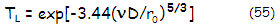and for short exposure by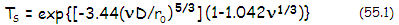where exp(...) is (...), being the natural logarithm base, 2.718 rounded to three decimals, and ν is the normalized spatial frequency.

It is important to note that the short exposure relation is not accurate for higher frequencies, for v~0.5 and larger. It produces higher contrast transfer in mid to high frequency range. It also produces an upward contrast spike between 0.9 and 1 frequency, becoming exponentially more pronounced as D/r0 value increases beyond ~5 (as Mahajan states, it is the consequence of assuming no correlation between tilt and roughness component of the seeing error, which is not strictly correct). The worst part of this high-frequency error - contrast spike up between 0.9 and 1 frequency - is effectively removed simply by using (1-ν1/3) instead of (1-1.042ν1/3). However, it still leaves in somewhat higher contrast in the mid to high frequency range. It can be nearly corrected by further lowering the constant in front of ν1/3 slightly below 1.

Combined atmospheric and telescope contrast transfer is given by the product of their respective OTF's. It is the final OTF in the image plane of the objective. Obstructed apertures will have lower combined OTF due to the contrast-lowering effect of central obstruction, but not more. In fact, the larger obstruction, the larger relative size of r0 vs. annulus, resulting in the slower increase in phase variance over annulus area and, consequently, slower decrease in the average Strehl (as a measure of wavefront quality, independent of the effect of central obstruction). This beneficial effect partly offsets the negative diffraction effect of central obstruction.

Effect of seeing on contrast transfer of a clear aberration-free aperture is given by a product the seeing OTF and Eq. 57, as shown below.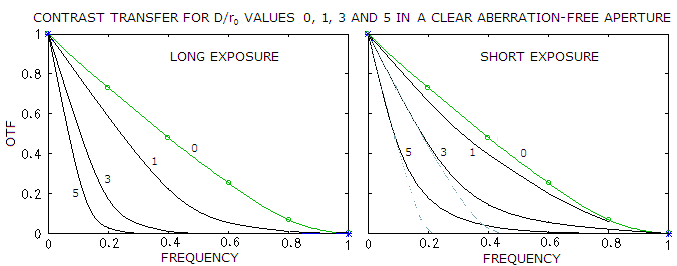FIGURE 87
: TOP: Long and short exposure seeing MTF in aberration-free aperture for selected
D/r0 values. The short exposure expression uses (1-ν1/3) instead of (1-1.042ν1/3), so somewhat higher than accurate contrast transfer in the mid to high frequencies is still present.
BOTTOM: Comparative plot for long exposure, short exposure and visual seeing MTF. The visual MTF has the modulating factor at right in the MTF relation adjusted so that it reflects the empirically based principle of the visual seeing error shifting from being nearly identical to short exposure seeing error for
D/r0~1 and smaller, gradually shifting toward being nearly identical to long exposure image for D/r~5 and larger (in order to remove small residual mid-to-high frequency range bias upward, short exposure plots for D/r0=2, 3, 5 and 10 were slightly modified by using 0.99, 0.97, 0.95 and 0.95 for the constant in front of ν1/3). As such, it is only approximate, but should give a good big picture of hao the visual MTF changes with D/r0.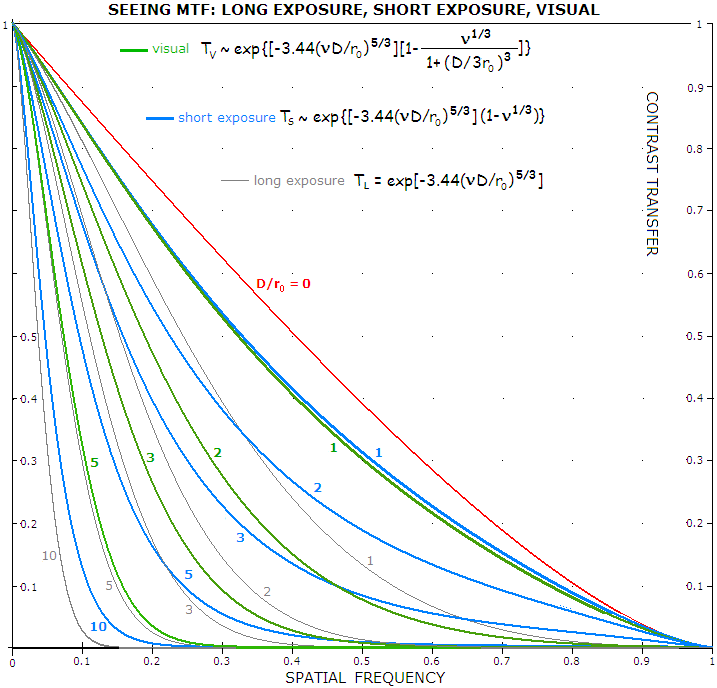The OTF plots show that, unlike classical aberrations, contrast drop caused by relatively significant seeing errors continues to increase toward high frequencies, without partial recovery in mid frequencies. This is a consequence of the expanding central maxima, which is the primary form of energy transfer with the seeing error (as opposed to non-random, classical aberrations, which mainly transfer energy to the rings, without significantly affecting size of the central maxima). It also causes loss of resolution sooner than classical aberrations that would result in similar contrast level in the lower range of frequencies.

Looking at the short exposure graph, as more representative of the visual seeing error in amateur-size apertures, it is evident that, contrast-wise, larger apertures tend to remain ahead of the smallest ones. The pattern is similar to that for stellar resolution. A 3 times larger aperture has the cutoff (zero contrast) projected from its mid-to-low frequencies (dashed line) still about 45% of the full resolving range for this aperture size, and 5 times larger aperture about 22% of the full resolving range for its aperture size. Adjusting for normalization (effectively, by compressing the plot horizontally by a ratio smaller-to-larger aperture diameter, i.e. smaller-to-larger D/r0 value), the 3 times larger aperture acts as about 30% larger aperture, contrast-wise, than the unit (D/r0=1) aperture, and 5 times larger aperture as about 10% larger (note that both larger apertures do maintain significant contrast well beyond the cutoff frequency of the smaller unit aperture, which are here 0.33 and 0.2, respectively, as well as beyond their projected cutoffs). This directly implies that 3 times larger aperture has better contrast level than either significantly smaller, or significantly larger apertures.

However, looking at the approximate visual plots, they are already closer to their respective long-exposure plots for D/r~3, and nearly coinciding with them for D/r~5 an larger. It makes improbable that five times larger aperture has any significant advantage in contrast transfer over the one for which D/r~1. Those 2 to 3 times larger are still better, and represent the best aperture range with respect to contrast transfer.

The discrepancy between the optimum aperture diameter being about 2r0 for limiting stellar resolution, and 2-3r0 for contrast transfer should be mainly the consequence of the short-exposure MTF becoming less representative of the actual visual MTF as D/r0 increases to ~2 and beyond, due to the increase in the effective magnitude of the tilt error component. In other words, their actual MTF plots are shifted toward the long-exposure plots, the larger D/r0, the more so. Consequently, the optimum D/r0 shifts toward lower value, in this case 2.

However, considering that seeing fluctuates, and that it will always be better than the average within appreciable portion of time, an optimum aperture, which would perform best during those times, should be somewhat larger than 2r0. Assuming that, according to the simplistic scheme on FIG. 82, seeing is likely to be 25% or more better than the average in about 1/4 of the time, the optimum aperture would be about 25% larger, or about 2.5r0. Factoring in those relatively frequent nights when the seeing gets better than typical would increase it to about 3r0.

Since it is the contrast level that determines resolution limit to extended details, like those on planetary surface, this implies that larger apertures, in general, outperform smallest ones in this respect, despite having a larger seeing error. At the same time, a range of apertures between really large and small apertures offer best performance level.

As the magnitude of seeing error increases, the visual seeing error shifts closer to the long exposure mode (FIG. 87, bottom). As the graph at left shows, even in the full  long-exposure mode (unlikely to occur in visual observing), loss of contrast transfer in larger apertures tends to be roughly offset by their size. In other words, contrast level remains similar in wide range of apertures. Since the actual visual seeing is between short- and long-exposure mode, the implication is that larger apertures tend to retain edge in contrast-related resolution, with the optimum aperture size being somewhere between small (D/r0~1) and large ones (D/r0~5, or more).

Closer to the observer, it is terrain topography, immediate surroundings and the very parts of a telescope itself that can create layers of unsteady air resulting in wavefront deformations.

5.1.1.1. Seeing and aperture       5.2. Low-level turbulence, tube currents...